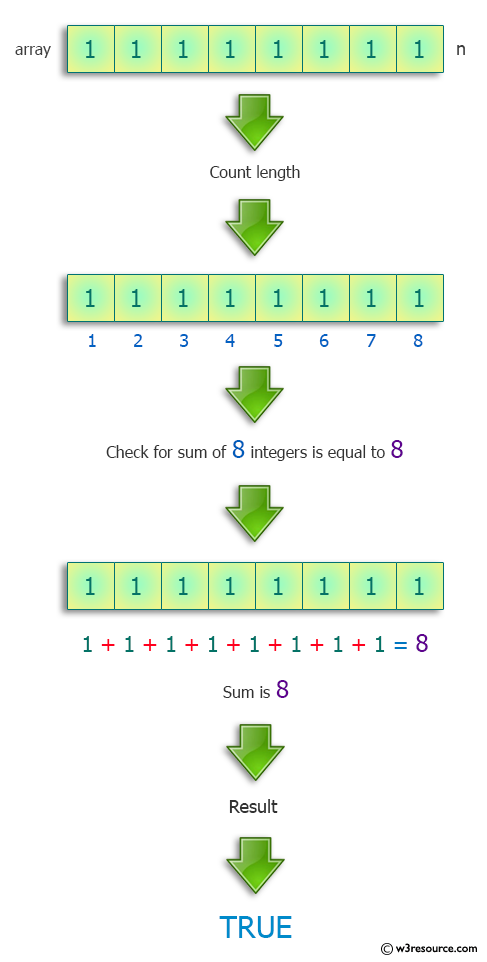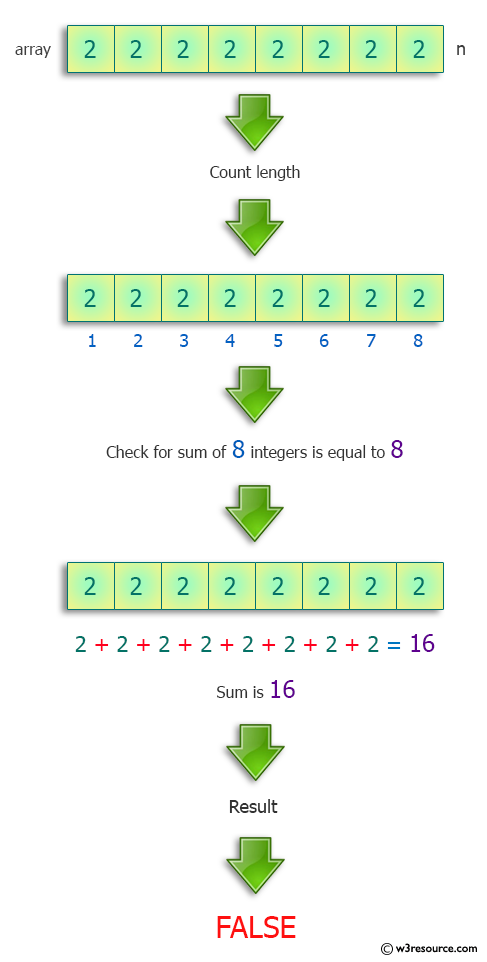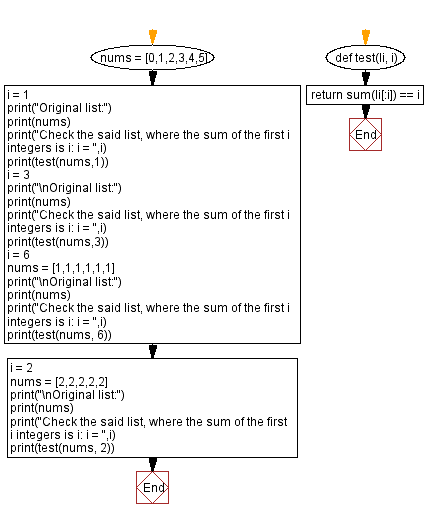﻿ Python: List of integers where the sum of the first i integers is i - w3resource# Python: List of integers where the sum of the first i integers is i

## Python Programming Puzzles: Exercise-7 with Solution

Write a Python program to check a given list of integers where the sum of the first i integers is i.

```Input:
[0, 1, 2, 3, 4, 5]
Output:
False

Input:
[1, 1, 1, 1, 1, 1]
Output:
True

Input:
[2, 2, 2, 2, 2]
Output:
False
```

Pictorial Presentation:Sample Solution:

Python Code:

``````#License: https://bit.ly/3oLErEI

def test(nums):
return all([sum(nums[:i]) == i for i in range(len(nums))])
nums = [0,1,2,3,4,5]
print("Original list:")
print(nums)
print("Check the said list, where the sum of the first i integers is i:")
print(test(nums))
nums = [1,1,1,1,1,1]
print("\nOriginal list:")
print(nums)
print("Check the said list, where the sum of the first i integers is i:")
print(test(nums))
nums = [2,2,2,2,2]
print("\nOriginal list:")
print(nums)
print("Check the said list, where the sum of the first i integers is i:")
print(test(nums))
``````

Sample Output:

```Original list:
[0, 1, 2, 3, 4, 5]
Check the said list, where the sum of the first i integers is i:
False

Original list:
[1, 1, 1, 1, 1, 1]
Check the said list, where the sum of the first i integers is i:
True

Original list:
[2, 2, 2, 2, 2]
Check the said list, where the sum of the first i integers is i:
False
```

Flowchart:## Visualize Python code execution:

The following tool visualize what the computer is doing step-by-step as it executes the said program:

Python Code Editor :

Have another way to solve this solution? Contribute your code (and comments) through Disqus.

What is the difficulty level of this exercise?

Test your Programming skills with w3resource's quiz.

﻿

## Python: Tips of the Day

Clamps num within the inclusive range specified by the boundary values x and y:

Example:

```def tips_clamp_num(num,x,y):
return max(min(num, max(x, y)), min(x, y))
print(tips_clamp_num(2, 4, 6))
print(tips_clamp_num(1, -1, -6))
```

Output:

```4
-1
```